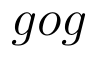Select Page

# Maths 12 Science Probability CBSE Answers for MCQ in English

Maths 12 Science Probability CBSE Answers for MCQ in English to enable students to get Answers in a narrative video format for the specific question.

Expert Teacher provides Maths 12 Science Probability CBSE Answers for MCQ through Video Answers in English language. This video solution will be useful for students to understand how to write an answer in exam in order to score more marks. This teacher uses a narrative style for a question from Probability not only to explain the proper method of answering question, but deriving right answer too.

Please find the question below and view the Answer in a narrative video format.

Question:

## Similar Questions from CBSE, 12th Science, Maths, Probability

Question 1 : One card is drawn from a pack of 52 cards. Find the probability of getting a jack of hearts:

Question 2 : In a game, a man wins rupee five for number six and losses rupee one for any other number, when a fair die is thrown. The man decided to throw a die thrice but to quit as and when he gets a six. Find the expected value of the amount he wins/losses. (View Answer Video)

Question 3 : Ifandthen find(View Answer Video)

Question 4 : In a hockey match, both teams A and B scored same number of goals up to the end of the game, so to decide the winner, the referee asked both the captains to throw a die alternately and decided that the team, whose captain gets a six first, will be declared the winner. If the captain of the team A was asked to start, find their respective probabilities of winning the match and state whether the decision of the referee was fair or not.      (View Answer Video)

Question 5 : A bag contains (2n+1) coins. It is known that (n-1) of these coins have a head on both sides, whereas the rest of the coins are fair. A coin is picked up at random from the bag and is tossed. If the probability that the toss results in a head is, determine the value of n. (View Answer Video)

### Differential Equations

Question 1 : Solve the following differential equation :(View Answer Video)

Question 2 : Find the particular solution of the differential equationgiven thatwhere x = 1. (View Answer Video)

Question 3 : Find the particular solution of the differential equationgiven that y = 0 when x = 1. (View Answer Video)

Question 4 : Write the degree of the differential equation :(View Answer Video)

### Continuity and Differentiability

Question 1 :  Find the second order derivative of the function. (View Answer Video)

Question 2 : Differentiate w.r.t.x the function. (View Answer Video)

Question 3 : Differentiate the function w.r.t.x. (View Answer Video)

Question 4 : Differentiate w.r.t.x the function, for some fixed a > 0 and x > 0. (View Answer Video)

Question 5 : Findfor the function. (View Answer Video)

### Relations and Functions

Question 1 : If f is the greatest integer function and g is the modulus function . Write the value of g o f(-1/3) – f o g ( -1/3 ). (View Answer Video)

Question 2 :  Letdefined as f(x) = 5 be a constant function. Then its range is (View Answer Video)

Question 3 : Functionsare defined respectively, by, find(View Answer Video)

Question 4 : Letbe defined as f(x) = 3x.  (View Answer Video)

Question 5 : Let A ={1, 2, 3}. Then number of equivalence relations containing (1, 2) is: (View Answer Video)